# How to Average Only Positive or Negative Numbers of a Range

Suppose both positive numbers and negative numbers exist in a table. If we want to know the average of only positive numbers in this table, we can create a formula to get average of all positive numbers with all negative numbers ignored. In this article, we will help you to construct a formula with AVERAGE and IF functions to get average of only positive numbers or negative numbers.

## EXAMPLE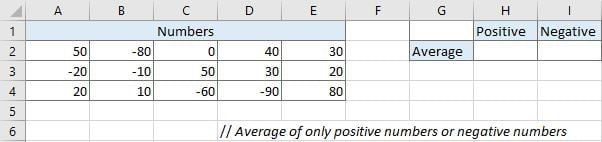Refer to above left side table, we can see both positive numbers and negative numbers are listed in range A2:E4. We want to calculate average of positive numbers and negative numbers separately and save results in H2 and I2 correspondingly.

In this instance, we will enter below formula into H2. We build this formula with AVERAGE and IF function.

`=IF(logical_test,[value_if_true],[value_if_false])`

## STEPS

nctions. The two functions are used frequently in Excel when running mathematics and logical expression.

## FUNCTION INTRODUCTION

a. AVERAGE function returns the average of numbers from a given range reference.

Syntax:

` =AVERAGE(number1, [number2], …)`

b. IF function returns “true value” or “false value” based on the result of provided logical test. It is one of the most popular function in Excel.

Syntax:a. In cell H2, enter the formula =AVERAGE(IF(A2:E4>0,A2:E4,””)). Range reference A2:E4 represents all numbers in this range.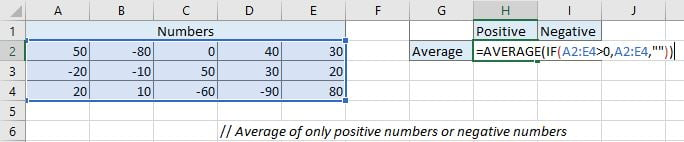This formula will execute IF function firstly to filter and keep all positive numbers in current array. If numbers are greater than 0, they are positive numbers. If logical expression “A2:E4>0” is true (number in A2:E4 is greater than 0), this number will be saved in array A2:E4.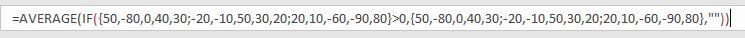After comparing each number in range A2:E4 with 0, below numbers are filtered and saved. Then AVERAGE function will calculate the average of these numbers.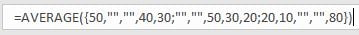b. After entering the formula, press Ctrl + Shift + Enter to load result because this is an array formula. But on Excel 365 you can directly press Enter as usual to load result.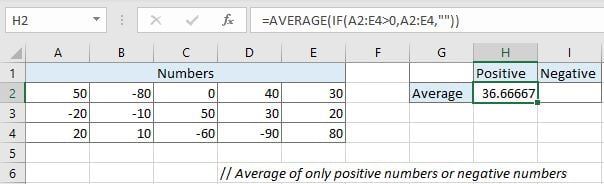c. In I2, enter the formula =AVERAGE(IF(A2:E4<0,A2:E4,””)). Press Ctrl + Shift + Enter to load result.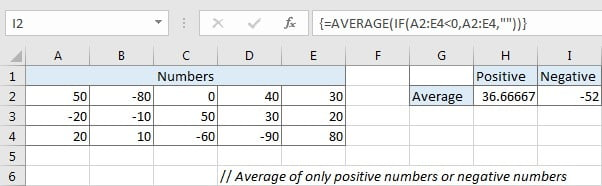### Related Functions

• Excel IF function
The Excel IF function perform a logical test to return one value if the condition is TRUE and return another value if the condition is FALSE. The IF function is a build-in function in Microsoft Excel and it is categorized as a Logical Function.The syntax of the IF function is as below:= IF (condition, [true_value],
• Excel AVERAGE function
The Excel AVERAGE function returns the average of the numbers that you provided.The syntax of the AVERAGE function is as below:=AVERAGE (number1,[number2],…)….
Related Posts

Calculate Days Open in Excel

If you want to know how to Calculate days in Excel, there are some formulas that you can use to do so. For example, you can use the DAYS function in Excel to find the number of days between two ...

Add Row Numbers And Skip Blanks in Excel

Do you ever have to input a list of numbers into a spreadsheet, and some of the cells are blank? It can be difficult to keep track of what number is in which cell when you have to scroll up ...

Assigning Points based on Late Time

It is shown in this lesson how to utilize the IF function in Excel to allocate points based on the amount of time that has passed. If you intend to pursue along this guide, you may do so by downloading ...

Check Dates in chronological order

Assume you have a date list that has different date formats, as seen in the accompanying picture. In this instance, Excel's Sort function will fail to sort them appropriately. However, you may convert all various date formats to a particular ...

Check If a Cell is Blank or Empty

The article demonstrates how to check if a range of cells is blank or empty by ISBLANK and other functions to recognize empty cells in Excel and take appropriate action based on their status. There are several circumstances in which ...

Abbreviate Names Or Words in Excel

As an MS Excel user, you might have come across a task where you need to abbreviate different names or words, and there are also possibilities that you might have done this task manually by assuming that there isn't any ...

Extract or Filter on The Top N Values with Criteria

Suppose that you are in a situation where you need to filter out the top n values from the list having few values with specific criteria, or you can say that with a particular condition, and I am also pretty ...

Extract matching values From Two Lists

Suppose that you are working with two lists containing few values, and you want to extract the matching values from those two lists into another separate list. You might prefer to manually extract the matching values from the two lists, ...

Extract Multiple Match Values into Separate Columns

If you have a few values/items in the excel sheet and you are thinking that with the aid of the “VlOOKUP” function you can look for a specific value, extract it and then put the matching item into the separate ...

VLOOKUP Formula | Faster Trick with 2 VLOOKUPS

This post will guide you how to use 2 VLOOKUPS function to looking up data entries from a given range of cells in Microsoft Excel. VLOOKUP with 2 lookups can be faster than a single VLOOKUP in certain scenarios. The ...

Sidebar